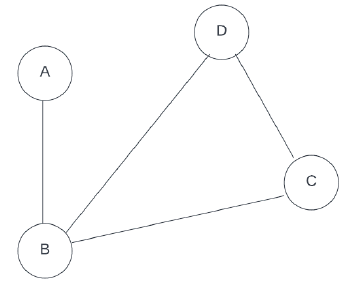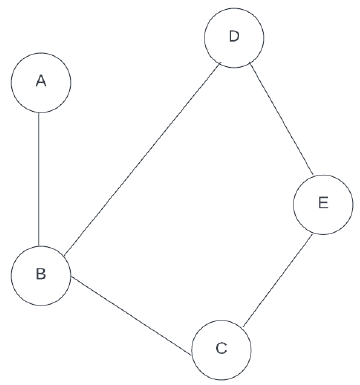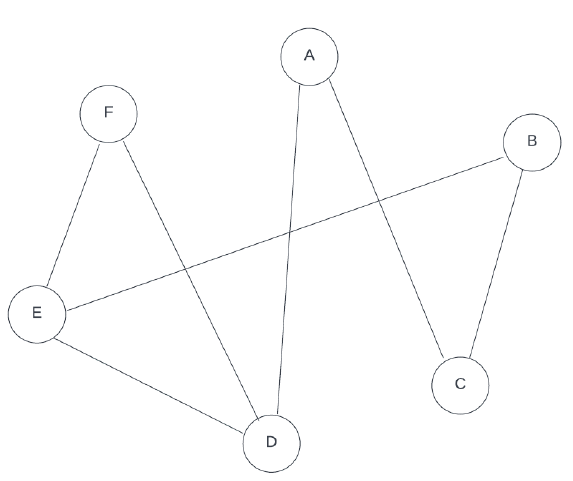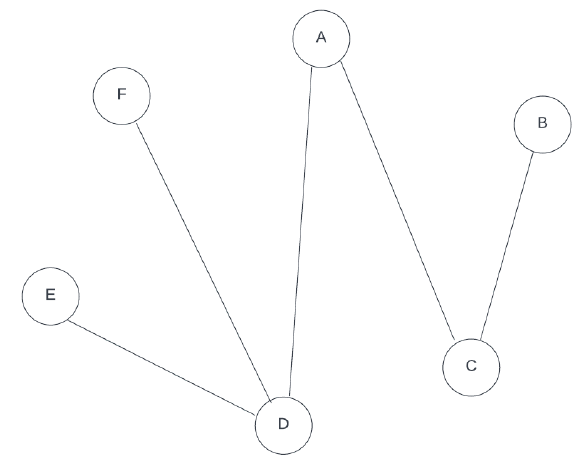# NPTEL Social Networks Assignment Answer (week 1-2) 2023

## NPTEL Social Networks Assignment Answer week 1 2023

1. If there exist a graph where nodes represents students and edges represents friendship, then for a rumour to be spread across entire class –

• Every student must know every other student.
• The graph needs to be connected.
• The graph need not be connected.
• Will spread in any case.
```Answer :- The graph needs to be connected.
```

2. If x = random.randrange(5,10), which values can x take?

• I) 5
• II) 8
• III) 4
• IV) 10
• Only I, II
• Only I, III
• Only I, III, IV
• Only I
`Answer :- only i , ii`

3. If x = random.randint(3,6), which values can x take?

I) 5

II) 4.3

III) 3

IV) 6

• Only I, II
• Only I, III
• Only I, III, IV
• Only I
`Answer :- Only I, III, IV`

4. What will be the output of the following code snippet?

x = [5, 2, 7, 3, 8]

try:

a = x

if(a%2 == 0):

print(“It is an even number”)

else:

print(“It is an odd number”)

except:

print(“Element does not exist”)

• It is an even number
• It is an odd number
• Element does not exist
• The code won’t run
`Answer :- Element does not exist`

5. What will be the output of the following code snippet?

import random

x = []

for i in range(7):

x.append(random.randint(1,5))

x.sort()

x.append({“one”:1, “two”:2})

print(len(x))

• 9
• 8
• 7
• 10
`Answer :- 8`

6. Maximum number of edges that can be present in a graph with 10 nodes are –

• 100
• 45
• 50
• 55
`Answer :- 45`

7. For a complete graph Z with 5 nodes if A=z.order()/z.size(), what will be the value of A?

• 1/4
• 1/8
• 1/2
• 1/16
`Answer :- 1/2`

8. What will nx.dijktra_path(G,u,v) return?

• Returns shortest path from u to v in a weighted graph
• Returns shortest path length
• Returns all possible paths from u to v
• Returns no. of possible paths from u to v
`Answer :- Returns shortest path from u to v in a weighted graph`

9. What will nx.gnp_random_graph(20,0.5) return?

• Returns graph with 20 nodes with half of the nodes connected.
• Returns graph with 20 nodes with each edge to be put with probability 0.5
• Returns a connected graph with 10 nodes.
• Returns a graph with 10 nodes with each edge to be put with probability 0.5
`Answer :- Returns graph with 20 nodes with each edge to be put with probability 0.5`

10. Maximum number of graphs possible from 50 nodes are –

• 50∗50
• 2(50/2)
• 50/2
• 5050
`Answer :- 2(50/2)`

## NPTEL Social Networks Assignment Answer week 2 2023

1. For graph G, what will the following code snippet return?

values = nx.degree(G).values()

x =0

for value in values:

if(x<value):

x = value

return x

• Returns the number of nodes with the minimum degree.
• Returns the number of nodes with the maximum degree.
• Returns the minimum degree of the graph.
• Returns the maximum degree of the graph.
`Answer :- Returns the maximum degree of the graph.`

2.The density of the given graph above is?

• 4/3
• 2/3
• 1/3
• 3/4
`Answer :- 2/3`

3. For the given graph, If A=Highest degree/∑degree, what will be the value of A?• 3/5
• 2/5
• 2/15
• 3/10
`Answer :- 3/10`

4. Which of the following is an example of a Directed graph?

I.   Network of Instagram followers

II.  Ancestral Tree

III. Email network

• Only IV
• Only I, II
• Only II, III
• Only I, II, III
`Answer :- Only I, II, III`

5.If X=clustering coefficient of node Eclustering coefficient of nodeF𝑋=𝑐𝑙𝑢𝑠𝑡𝑒𝑟𝑖𝑛𝑔 𝑐𝑜𝑒𝑓𝑓𝑖𝑐𝑖𝑒𝑛𝑡 𝑜𝑓 𝑛𝑜𝑑𝑒 𝐸𝑐𝑙𝑢𝑠𝑡𝑒𝑟𝑖𝑛𝑔 𝑐𝑜𝑒𝑓𝑓𝑖𝑐𝑖𝑒𝑛𝑡 𝑜𝑓 𝑛𝑜𝑑𝑒𝐹 in the given graph, the value of X is ___.• 1
• 1/3
• 1/9
• 2/3
`Answer :- 1/3`

6. Which of the following is/are network dataset format?

I.   GraphML

II.  Pajek NET

III. Comma Separated Value(Edge List format)

• Only II
• Only III
• Only I, III
• Only I, II, III
`Answer :- Only I, II, III`

7. In graph G, where nodes represent words in a dictionary and there is an edge between two nodes if the two words are synonymous. Then, choose the correct option according to the given two statements.

Statement I: The graph G is connected.
Statement II: If the word A is connected to B & B is connected to C, then A is synonymous to C.

• Both statements are incorrect.
• Statement I is incorrect & Statement II is correct.
• Statement I is correct & Statement II is incorrect.
• Both statements are correct.
```Answer :- Statement I is correct & Statement II is incorrect.
```

8. The diameter of the given graph G is ___.• 5
• 4
• 3
• 2
`Answer :- 4`

9. If there exist 𝑛 nodes with no edges initially then, what is the probability of node V being isolated after including 𝑛𝑙𝑜𝑔(𝑛) edges uniformly at random?

• 1/e
• 1/nlog(n)
• 1/n
• 1/n2
`Answer :- 1/n2`

10. Choose the data set format which starts with the keyword “graph”?

• GML
• Graph Exchange XML
• Pajek Net format
• GEXF
`Answer :- GML`
Scroll to Top